Updating search results...# Grade 4 DLC Blended Math

This collection features resources developed by The Distance Learning Centre (DLC) for blended math in Grade 4.

76 affiliated resources

# Search Resources

View
Selected filters: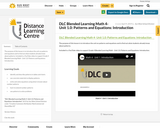Conditional Remix & Share Permitted
CC BY-NC-SA
Rating
0.0 stars

The purpose of this lesson is to introduce the unit on patterns and equations and to find out what students already know about patterns.

Included is a YouTube video to support Grade 4 Blended Learning Math - Unit 1.0: Patterns and Equations: Introduction.

Subject:
Math
Material Type:
Activity/Lab
Homework/Assignment
Lesson
Provider:
Sun West Distance Learning Centre (DLC)
04/09/2019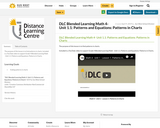Conditional Remix & Share Permitted
CC BY-NC-SA
Rating
0.0 stars

The purpose of this lesson is to find patterns in charts.

Included is a YouTube video to support Grade 4 Blended Learning Math - Unit 1.1: Patterns and Equations: Patterns in Charts.

Subject:
Math
Material Type:
Activity/Lab
Homework/Assignment
Lesson
Provider:
Sun West Distance Learning Centre (DLC)
04/09/2019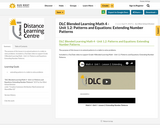Conditional Remix & Share Permitted
CC BY-NC-SA
Rating
0.0 stars

The purpose of this lesson is to extend patterns in a table to solve problems.

Included is a YouTube video to support Grade 4 Blended Learning Math - Unit 1.2: Patterns and Equations: Extending Number Patterns.

Subject:
Math
Material Type:
Activity/Lab
Homework/Assignment
Lesson
Provider:
Sun West Distance Learning Centre (DLC)
04/09/2019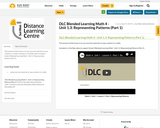Conditional Remix & Share Permitted
CC BY-NC-SA
Rating
0.0 stars

The purpose of this lesson is to use concrete materials to copy a pattern in a table.

Included is a YouTube video to support Grade 4 Blended Learning Math - Unit 1.3: Representing Patterns (Part 1).

Subject:
Math
Material Type:
Activity/Lab
Homework/Assignment
Lesson
Provider:
Sun West Distance Learning Centre (DLC)
04/09/2019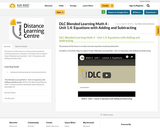Conditional Remix & Share Permitted
CC BY-NC-SA
Rating
0.0 stars

The purpose of this lesson is to make and solve equations involving subtraction.

Included is a YouTube video to support Grade 4 Blended Learning Math - Unit 1.4: Equations with Adding and Subtracting.

Subject:
Math
Material Type:
Activity/Lab
Homework/Assignment
Lesson
Provider:
Sun West Distance Learning Centre (DLC)
04/09/2019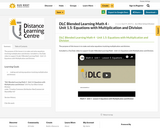Conditional Remix & Share Permitted
CC BY-NC-SA
Rating
0.0 stars

The purpose of this lesson is to make and solve equations involving multiplication and division.

Included is a YouTube video to support Grade 4 Blended Learning Math - Unit 1.5: Equations with Multiplication and Division.

Subject:
Math
Material Type:
Activity/Lab
Homework/Assignment
Lesson
Provider:
Sun West Distance Learning Centre (DLC)
04/09/2019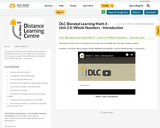Conditional Remix & Share Permitted
CC BY-NC-SA
Rating
0.0 stars

The purpose of this lesson is to introduce and activate prior knowledge about the topic of whole numbers.

Included is a YouTube video to support Grade 4 Blended Learning Math - Unit 2.0: Whole Numbers - Introduction.

Subject:
Math
Material Type:
Activity/Lab
Homework/Assignment
Lesson
Provider:
Sun West Distance Learning Centre (DLC)
04/09/2019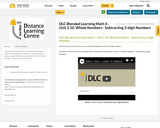Conditional Remix & Share Permitted
CC BY-NC-SA
Rating
0.0 stars

The purpose of this lesson is to use personal strategies to subtract 3-digit numbers.

Included is a YouTube video to support Grade 4 Blended Learning Math - Unit 2.10: Whole Numbers - Subtracting 3-digit Numbers.

Subject:
Math
Material Type:
Activity/Lab
Homework/Assignment
Lesson
Provider:
Sun West Distance Learning Centre (DLC)
04/17/2019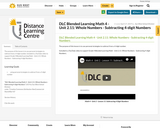Conditional Remix & Share Permitted
CC BY-NC-SA
Rating
0.0 stars

The purpose of this lesson is to use personal strategies to subtract from a 4-digit number.

Included is a YouTube video to support Grade 4 Blended Learning Math - Unit 2.11: Whole Numbers - Subtracting 4-digit Numbers.

Subject:
Math
Material Type:
Activity/Lab
Homework/Assignment
Lesson
Provider:
Sun West Distance Learning Centre (DLC)
04/17/2019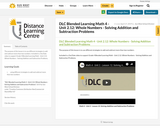Conditional Remix & Share Permitted
CC BY-NC-SA
Rating
0.0 stars

The purpose of this lesson is to use different strategies to add and subtract more than two numbers.

Included is a YouTube video to support Grade 4 Blended Learning Math - Unit 2.12: Whole Numbers - Solving Addition and Subtraction Problems.

Subject:
Math
Material Type:
Activity/Lab
Homework/Assignment
Lesson
Provider:
Sun West Distance Learning Centre (DLC)
04/17/2019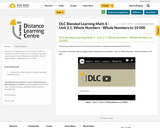Conditional Remix & Share Permitted
CC BY-NC-SA
Rating
0.0 stars

The purpose of this lesson is to read and write numbers in standard, expanded and word forms.

Included is a YouTube video to support Grade 4 Blended Learning Math - Unit 2.1: Whole Numbers - Whole Numbers to 10 000.

Subject:
Math
Material Type:
Activity/Lab
Homework/Assignment
Lesson
Provider:
Sun West Distance Learning Centre (DLC)
04/09/2019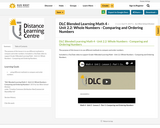Conditional Remix & Share Permitted
CC BY-NC-SA
Rating
0.0 stars

The purpose of this lesson is to use different methods to compare and order numbers.

Included is a YouTube video to support Grade 4 Blended Learning Math - Unit 2.2: Whole Numbers - Comparing and Ordering Numbers.

Subject:
Math
Material Type:
Activity/Lab
Homework/Assignment
Lesson
Provider:
Sun West Distance Learning Centre (DLC)
04/17/2019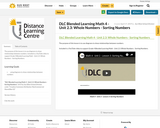Conditional Remix & Share Permitted
CC BY-NC-SA
Rating
0.0 stars

The purpose of this lesson is to use diagrams to show relationships between numbers.

Included is a YouTube video to support Grade 4 Blended Learning Math - Unit 2.3: Whole Numbers - Sorting Numbers.

Subject:
Math
Material Type:
Activity/Lab
Homework/Assignment
Lesson
Provider:
Sun West Distance Learning Centre (DLC)
04/17/2019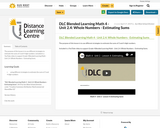Conditional Remix & Share Permitted
CC BY-NC-SA
Rating
0.0 stars

The purpose of this lesson is to use different strategies to estimate the sums of 3 and 4-digit numbers.

Included is a YouTube video to support Grade 4 Blended Learning Math - Unit 2.4: Whole Numbers - Estimating Sums.

Subject:
Math
Material Type:
Activity/Lab
Homework/Assignment
Lesson
Provider:
Sun West Distance Learning Centre (DLC)
04/17/2019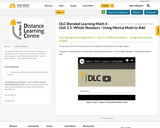Conditional Remix & Share Permitted
CC BY-NC-SA
Rating
0.0 stars

The purpose of this lesson is to use personal strategies to mentally add 3 and 4-digit numbers.

Included is a YouTube video to support Grade 4 Blended Learning Math - Unit 2.5: Whole Numbers - Using Mental Math to Add.

Subject:
Math
Material Type:
Activity/Lab
Homework/Assignment
Lesson
Provider:
Sun West Distance Learning Centre (DLC)
04/17/2019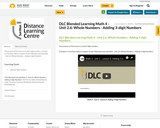Conditional Remix & Share Permitted
CC BY-NC-SA
Rating
0.0 stars

The purpose of this lesson is to add 3-digit numbers.

Included is a YouTube video to support Grade 4 Blended Learning Math - Unit 2.6: Whole Numbers - Adding 3-digit Numbers.

Subject:
Math
Material Type:
Activity/Lab
Homework/Assignment
Lesson
Provider:
Sun West Distance Learning Centre (DLC)
04/17/2019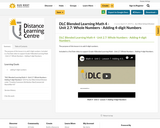Conditional Remix & Share Permitted
CC BY-NC-SA
Rating
0.0 stars

The purpose of this lesson is to add 4-digit numbers.

Included is a YouTube video to support Grade 4 Blended Learning Math - Unit 2.7: Whole Numbers - Adding 4-digit Numbers.

Subject:
Math
Material Type:
Activity/Lab
Homework/Assignment
Lesson
Provider:
Sun West Distance Learning Centre (DLC)
04/17/2019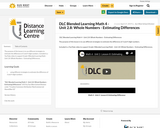Conditional Remix & Share Permitted
CC BY-NC-SA
Rating
0.0 stars

The purpose of this lesson is to use different strategies to estimate the difference of 3 and 4-digit numbers.

Included is a YouTube video to support Grade 4 Blended Learning Math - Unit 2.8: Whole Numbers - Estimating Differences.

Subject:
Math
Material Type:
Activity/Lab
Homework/Assignment
Lesson
Provider:
Sun West Distance Learning Centre (DLC)
04/17/2019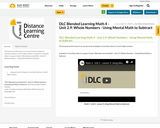Conditional Remix & Share Permitted
CC BY-NC-SA
Rating
0.0 stars

The purpose of this lesson is to use personal strategies to mentally subtract 3 and 4-digit numbers.

Included is a YouTube video to support Grade 4 Blended Learning Math - Unit 2.9: Whole Numbers - Using Mental Math to Subtract.

Subject:
Math
Material Type:
Activity/Lab
Homework/Assignment
Lesson
Provider:
Sun West Distance Learning Centre (DLC)
04/17/2019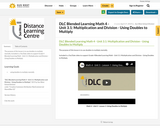Conditional Remix & Share Permitted
CC BY-NC-SA
Rating
0.0 stars

The purpose of this lesson is to use doubles to multiply mentally.

Included is a YouTube video to support Grade 4 Blended Learning Math - Unit 3.1: Multiplication and Division - Using Doubles to Multiply.

Subject:
Math
Material Type:
Activity/Lab
Homework/Assignment
Lesson
Provider:
Sun West Distance Learning Centre (DLC)